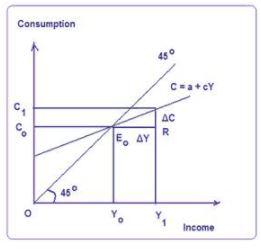Courses

# Consumption Function - Macroeconomics B Com Notes | EduRev

## Macro Economics

Created by: Arshit Thakur

## B Com : Consumption Function - Macroeconomics B Com Notes | EduRev

The document Consumption Function - Macroeconomics B Com Notes | EduRev is a part of the B Com Course Macro Economics.
All you need of B Com at this link: B Com

Keynes’ Consumption Function: The Absolute Income Hypothesis

Keynes in his General Theory postulated that aggregate consumption is a function of aggregate current disposal income. The relation between consumption and income is based on his fundamental psychological law of consumption which states that when income increases consumption expenditure also increases but by a smaller amount.

The Keynesian consumption function is written as

C = a + cY                  a > 0                0 < c < 1

Where a is the intercept, a constant which measures consumption at a zero level of disposal income, c is the marginal propensity to consume MPC and Y is the disposal income.

The above relation that consumption is a function of current disposable income whether linear or non-linear is called the absolute income hypothesis. This consumption function has the following properties:

1. As income increases, average propensity to consume (APC = C / Y) falls.
2. The marginal propensity to consume MPC is positive but less than unity (0 < C < 1) so that higher income leads to higher consumption.
3. The consumption expenditure increases or decreases with increase or decrease in income but non-proportionally. This non-proportional consumption function implies that in the short run average and marginal propensities do not coincide (APC > MPC).
4. This consumption function is stable both in the short run and the long run.This consumption function is expressed in diagram 1, where C = a + cY is the consumption function. At point E on the C curve the income level is OY1. At this point, APC > MPC where APC = OC1 / OY1 and MPC = ΔC / ΔY = ER / RE0. This shows disproportional consumption function. The intercept ‘a’ shows the level of consumption corresponding to a zero level of income.

At income level OY0 where the curve C intersects the 45 degree line, point E0 represents APC (=OC0 / OY0). Below the income level OY0, consumption is more than income. In this range, APC is greater than 1. Above the income level OY0 consumption increases less than proportionately with income so that APC declines and it is less than one.

Offer running on EduRev: Apply code STAYHOME200 to get INR 200 off on our premium plan EduRev Infinity!

,

,

,

,

,

,

,

,

,

,

,

,

,

,

,

,

,

,

,

,

,

;